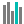#CHISQ.INV (PQL - xl)

This function returns the criterion binomial, the smallest value for which the cumulative binomial distribution is greater than or equal to a criterion value

• Library: PQL \ Spreadsheet \ Statistical
• Compatibility: Any content (regardless of data source) in the Tabulate spreadsheet module

#### Syntax

CHISQ.INV(probability, deg freedom)

##### Function Arguments
 Name Description Type Optional probability Probability of the chi-squared distribution Number deg freedom Number of degrees of freedom; if not an integer, the number is truncated Number